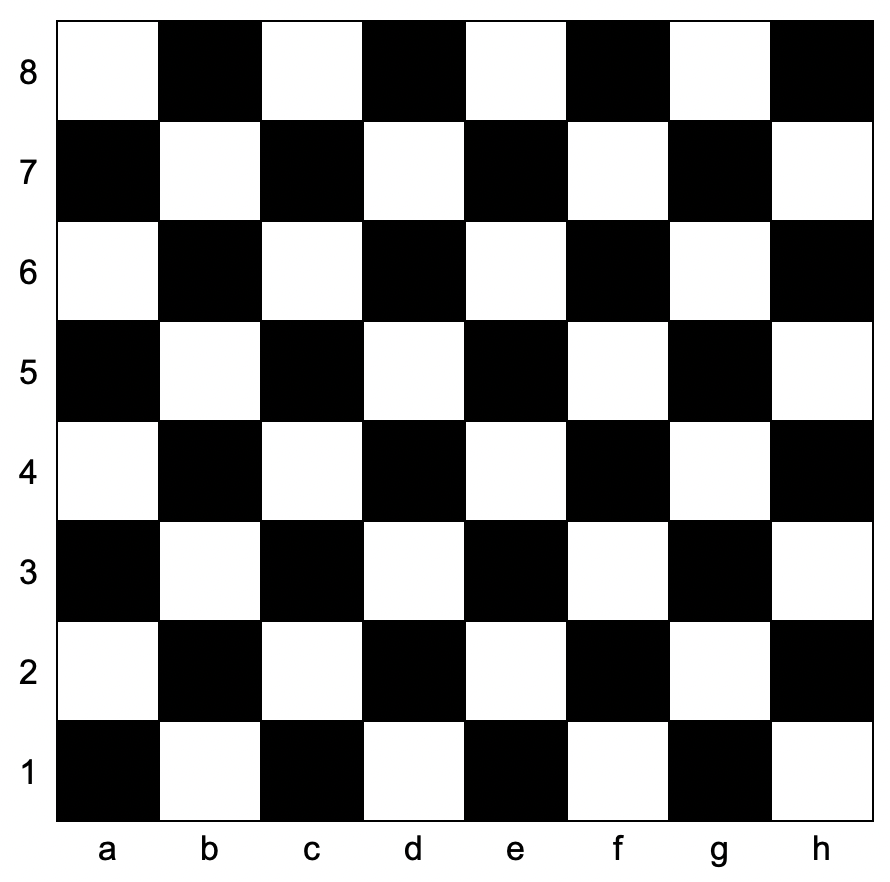Formatted question description: https://leetcode.ca/all/1812.html

# 1812. Determine Color of a Chessboard Square

Easy

## Description

You are given coordinates, a string that represents the coordinates of a square of the chessboard. Below is a chessboard for your reference.Return true if the square is white, and false if the square is black.

The coordinate will always represent a valid chessboard square. The coordinate will always have the letter first, and the number second.

Example 1:

Input: coordinates = “a1”

Output: false

Explanation: From the chessboard above, the square with coordinates “a1” is black, so return false.

Example 2:

Input: coordinates = “h3”

Output: true

Explanation: From the chessboard above, the square with coordinates “h3” is white, so return true.

Example 3:

Input: coordinates = “c7”

Output: false

Constraints:

• coordinates.length == 2
• 'a' <= coordinates <= 'h'
• '1' <= coordinates <= '8'

## Solution

Convert coordinates into two integers, which represent the column and the row of the square. Both the column and the row range from 1 to 8. The square is white if and only if the column is odd and the row is even, or the column is even and the row is odd.

class Solution {
public boolean squareIsWhite(String coordinates) {
int column = coordinates.charAt(0) - 'a' + 1;
int row = coordinates.charAt(1) - '0';
return column % 2 != row % 2;
}
}